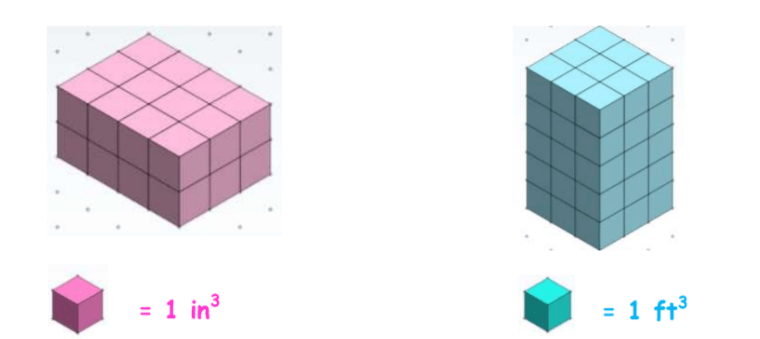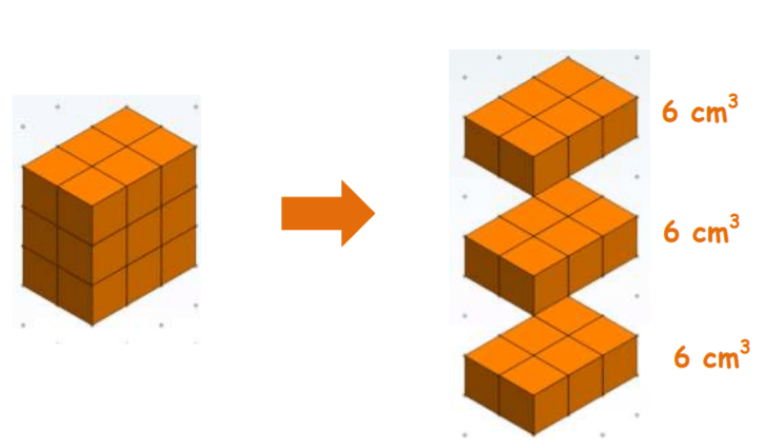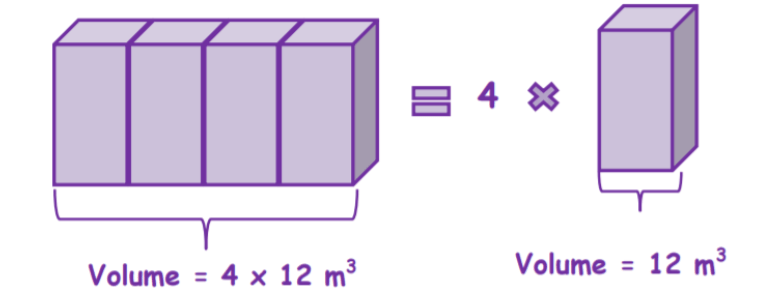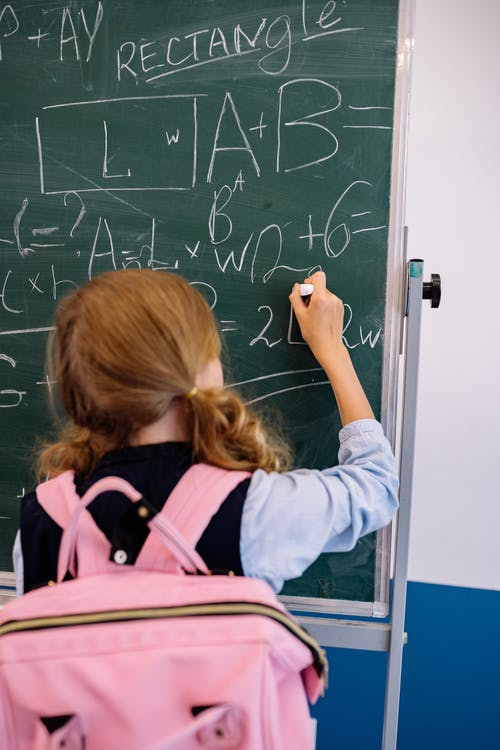# Measuring Volume

 Listen to this Lesson:

In units on measuring volume, fifth graders learn how to find the volume of one layer of a figure by counting the cubes, how to apply addition when finding the total volume of a figure, and how to use multiplication when finding the volume of a figure given the volume of one layer.

Whether you’re a math teacher at a school or a homeschooling parent, there are tons of strategies that you can adopt while teaching volume in grade 5. To help out, we bring you a few such strategies. Use them in your class and have children measuring volume in no time!## Strategies to Teach Measuring Volume

### What Is Volume?

Start your lesson on measuring volume by defining volume. Volume is a measurement of the capacity of objects. To have volume, an object must have the following dimensions: length, width, and height. Draw an example of an object that has these dimensions, such as:### Measuring Volume in Cubic Units

Explain that one of the most common three-dimensional figures used in volumes are cubes. The height, length, and depth of a cube are all equal. A cube with 1-cm dimensions has a volume of 1 cm3. The same thing goes for all other units of measure such as cm, in, m, dm, etc.

Provide examples of measuring volume in cubic units. For instance, draw two figures on the whiteboard, one rectangular prism with 24 cubes and another rectangular prism with 45 cubes. Write the measurement of one cube in each case, in inches or feet, such as:Explain that in the first case, the rectangular prism has 24 cubes where one cube equals 1 inch3, so the volume of the prism is 24 cubic inches. In the second case, the rectangular prism has 45 cubes where one cube equals 1 feet3 so the volume of the prism is 45 ft3.

### Measuring Volume by Adding Volume of Layers

Explain that volumes of three-dimensional figures can also be determined by adding up the volume of each layer. This is most helpful when the volume of each layer is given, or when it’s easier to count the layers first before finding the total volume of the figure.

Draw an example on the whiteboard to illustrate how we can measure volume by adding volume of layers. For instance, you can draw a rectangular prism with three layers, such as the prism below:Point out that since 1 cube has a volume of 1 in3, and there are 6 cubes in each layer, we can infer that one layer has a volume of 6 cm3. We can find the volume of the figure by adding up the volumes of the layers.

Thus, if each layer has a volume of 6 cm3, the total volume of the figure is:

6 cm3 + 6 cm3 + 6 cm3 = 18 cm3.

Point out that there are cases where we don’t need to calculate the volume of each layer, as it’s given beforehand, so the only thing left to do then is to determine the total volume by simply adding up the volume of layers.

Provide an example of a figure where the volume of layers is given. For instance:In this case, the only thing left to do to find the volume of the figure is to add the volume of the three different layers, which will result in the following: 15 in3 + 10 in3 + 22 in3 = 47 in3. So the total volume of the figure is 47 in3.

### Measuring Volume by Multiplication

Point out that there are some figures (for example, rectangular prisms) that have identical layers, so with these figures, it would be easier if we multiply the number of layers by the volume of one of the layers. We call this measuring volume by multiplication.

Provide an example of this. For instance, draw a figure on the whiteboard that has four identical layers and illustrate how to measure its volume by multiplication:Explain that since we know that one layer has a volume of 12 m3, a figure made up of four identical layers will have a volume of 4 × 12 = 48 m3. In other words, the total volume of the figure is 48 m3.

If you have the technical means in your classroom, you can also complement your lesson with multimedia materials, such as videos. Videos will be especially useful in helping students visualize the different figures.

For example, use this video to introduce the concept of volume and to make a comparison between volume, length, and area. Then, play this video to provide an introduction to measuring the volume of rectangular prisms with unit cubes.

This video is also a great resource on finding volume with unit cubes, illustrating how to measure the volume of rectangular prisms. Finally, to make the lesson more fun and engaging, use this video that represents a volume song.## Activities to Practice Measuring Volume

### Volume of Rectangular Prisms With Unit Cubes

This is a simple online game where students can practice finding the volume of rectangular prisms with unit cubes. To use this activity in your class, the only materials needed are technical devices for students.

Pair students up and provide instructions for the game. Students are presented with several exercises where they should measure the volume of a given rectangular prism in cubic units. They can add the volume of layers or use multiplication for this purpose.

Students play the game individually in each pair. If they get stuck, they can use a hint or watch a related video in the game. In the end, students compare their results and discuss how they solved the exercises with their partner in the pair.

### Prism Matching Game

This is a game where students get to practice using multiplication when finding the volume of a figure given the volume of one layer. To use this game in your class, you’ll need colorful construction paper, scissors, and some pencils.

Draw several prisms on the construction paper and make sure they have cubes inside them, as children will use them for finding the volume of the prism. For each prism that you draw, draw an additional prism with the same volume, but in a different color and a different position.

Cut the prisms and mix them up in a pile. Create several such piles, each containing 20 prism pairs. The number of piles will depend on the number of students in your class (there should be one pile for each group).

Divide students into groups of 3, 4 and hand out the prism piles. Provide instructions for the game. Students in each group try to measure the volume of the prisms in their pile and match them with a prism of a different color that has the same volume.

This is a fast-paced game, so the winner is the group that manages to match all prisms first. You can also use a timer to measure the time needed to complete the activity. Introduce small, symbolic prizes for the first three groups.

### Pair Work

This is a simple activity that will provide students with the opportunity to sharpen their skills at measuring the volume of a given figure. The only material needed to implement this activity in your classroom is this Assignment Worksheet (Members Only).

Pair students up and hand out the worksheet. Explain that the worksheet contains several exercises related to measuring volume (ex: determining which figure has a bigger volume when given the volume of each figure’s layer).

Students in each pair work together to complete the exercises, which will also allow them to engage in peer tutoring. This is why it’s important that students are paired up in an adequate way. Homeschooling parents can use the activity as an individual one.

In the end, open space for discussion and reflection within the class. How did students manage to find the volume in each exercise? Were there cases where they added up the volume of each layer? Were there cases with identical layers where they used multiplication to measure the volume of the given figure?

## Before You Leave…

If you enjoyed these strategies and activities for teaching measuring volume, you may want to check out our lesson that’s dedicated to this topic! So if you need guidance to structure your class and teach it, sign up for our emails to receive loads of free content!

Feel free to also check out our blog – you’ll find plenty of awesome resources that you can use in your class, such as this article with free activities and worksheets on teaching volume of a right rectangular prism!